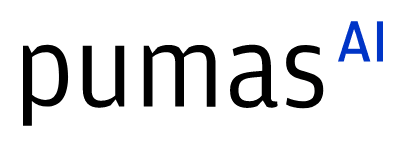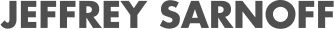# NExOS.jl for Nonconvex Exterior-point Operator Splitting

07/29/2021, 7:00 PM7:30 PM UTC
JuMP Track

#### Abstract:

NExOS.jl is a Julia package that implements the Nonconvex Exterior-point Operator Splitting (NExOS) algorithm (https://arxiv.org/pdf/2011.04552.pdf). The package is tailored for minimizing a convex cost function over a nonconvex constraint set, where projection onto the constraint set is single-valued around local minima.

#### Description:

We consider the problem of minimizing a convex cost function over a nonconvex constraint set, where projection onto the constraint set is single-valued around points of interest. A wide range of nonconvex learning problems have this structure including (but not limited to) sparse and low-rank optimization problems.

By exploiting the underlying geometry of the constraint set, NExOS finds a locally optimal point by solving a sequence of penalized problems with strictly decreasing penalty parameters. NExOS solves each penalized problem by applying an outer iteration operator splitting algorithm, which converges linearly to a local minimum of the corresponding penalized formulation under regularity conditions. Furthermore, the local minima of the penalized problems converge to a local minimum of the original problem as the penalty parameter goes to zero.

NExOS.jl has been extensively tested on many instances from a wide variety of learning problems. In spite of being general-purpose, NExOS is able to compute high-quality solutions very quickly and is competitive with specialized algorithms.